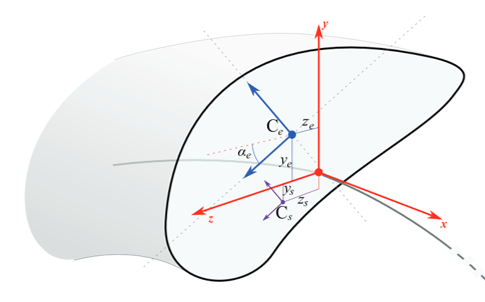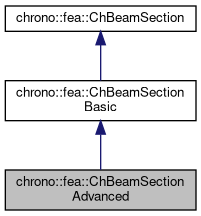## Description

Geometry for a beam section in 3D, along with basic material properties.

It also supports the advanced case of Iyy and Izz axes rotated respect reference, centroid with offset from reference, and shear center with offset from reference. This material can be shared between multiple beams.#include <ChBeamSection.h>[legend][legend]

## Public Member Functions

void SetSectionRotation (double ma)
Set the rotation of the section for which the Iyy Izz are defined.

double GetSectionRotation ()

void SetCentroid (double my, double mz)
Set the displacement of the centroid C (i.e. More...

double GetCentroidY ()

double GetCentroidZ ()

void SetShearCenter (double my, double mz)
Set the displacement of the shear center S with respect to the reference beam line. More...

double GetShearCenterY ()

double GetShearCenterZ ()Public Member Functions inherited from chrono::fea::ChBeamSectionBasic
void SetArea (const double ma)
Set the cross sectional area A of the beam (m^2)

double GetArea () const

void SetIyy (double ma)
Set the Iyy moment of inertia of the beam (for flexion about y axis) Note: some textbook calls this Iyy as Iz.

double GetIyy () const

void SetIzz (double ma)
Set the Izz moment of inertia of the beam (for flexion about z axis) Note: some textbook calls this Izz as Iy.

double GetIzz () const

void SetJ (double ma)
Set the J torsion constant of the beam (for torsion about x axis)

double GetJ () const

void SetKsy (double ma)
Set the Timoshenko shear coefficient Ks for y shear, usually about 0.8, (for elements that use this, ex. More...

double GetKsy () const

void SetKsz (double ma)
Set the Timoshenko shear coefficient Ks for z shear, usually about 0.8, (for elements that use this, ex. More...

double GetKsz () const

void SetAsRectangularSection (double width_y, double width_z)
Shortcut: set Area, Ixx, Iyy, Ksy, Ksz and J torsion constant at once, given the y and z widths of the beam assumed with rectangular shape.

void SetAsCircularSection (double diameter)
Shortcut: set Area, Ixx, Iyy, Ksy, Ksz and J torsion constant at once, given the diameter of the beam assumed with circular shape.

void SetDensity (double md)
Set the density of the beam (kg/m^3)

double GetDensity () const

void SetYoungModulus (double mE)
Set E, the Young elastic modulus (N/m^2)

double GetYoungModulus () const

void SetGshearModulus (double mG)
Set G, the shear modulus.

double GetGshearModulus () const

void SetGwithPoissonRatio (double mpoisson)
Set G, the shear modulus, given current E and the specified Poisson ratio.

void SetBeamRaleyghDamping (double mr)
Set the Rayleigh damping ratio r (as in: R = r * K ), to do: also mass-proportional term.

double GetBeamRaleyghDamping ()Public Member Functions inherited from chrono::fea::ChBeamSection
void SetDrawShape (std::shared_ptr< ChBeamSectionShape > mshape)
Set the graphical representation for this section. More...

std::shared_ptr< ChBeamSectionShapeGetDrawShape () const
Get the drawing shape of this section (i.e.a 2D profile used for drawing 3D tesselation and visualization) By default a thin square section, use SetDrawShape() to change it.

void SetDrawThickness (double thickness_y, double thickness_z)
Shortcut: adds a ChBeamSectionShapeRectangular for visualization as a centered rectangular beam, and sets its width/height. More...

Shortcut: adds a ChBeamSectionShapeCircular for visualization as a centered circular beam, and sets its radius. More...

void SetCircular (bool ic)
OBSOLETE only for backward compability More...

## Public Attributes

double alpha

double Cy

double Cz

double Sy

double SzPublic Attributes inherited from chrono::fea::ChBeamSectionBasic
double Area

double Iyy

double Izz

double J

double G

double E

double density

double rdamping

double Ks_y

double Ks_z

## ◆ SetCentroid()

 void chrono::fea::ChBeamSectionAdvanced::SetCentroid ( double my, double mz )
inline

Set the displacement of the centroid C (i.e.

the elastic center, or tension center) with respect to the reference beam line.

## ◆ SetShearCenter()

 void chrono::fea::ChBeamSectionAdvanced::SetShearCenter ( double my, double mz )
inline

Set the displacement of the shear center S with respect to the reference beam line.

For shapes like rectangles, rotated rectangles, etc., it corresponds to the centroid C, but for "L" shaped or "U" shaped beams this is not always true, and the shear center accounts for torsion effects when a shear force is applied.

The documentation for this class was generated from the following file:
• /builds/uwsbel/chrono/src/chrono/fea/ChBeamSection.h